PHP 基础

122 篇文章 24 订阅主要特点

（一）开源性和免费性

（二）快捷性

PHP是一种非常容易学习和使用的一门语言，它的语法特点类似于C语言，但又没有C语言复杂的地址操作，而且又加入了面向对象的概念，再加上它具有简洁的语法规则，使得它操作编辑非常简单，实用性很强。

（三）数据库连接的广泛性

PHP可以与很多主流的数据库建立起连接，如MySQLODBCOracle等，PHP是利用编译的不同函数与这些数据库建立起连接的，PHPLIB就是常用的为一般事务提供的基库。

（四）面向过程和面向对象并用

优点

（一）流行，容易上手

PHP是目前最流行的编程语言，这毋庸置疑。它驱动全球超过2亿多个网站，有全球超过81.7%的公共网站在服务器端采用PHP。PHP常用的数据结构都内置了，使用起来方便简单，也一点都不复杂，表达能力相当灵活。

（二）开发职位很多

（三）仍然在不断发展

PHP在不断兼容着类似closures和命名空间等技术，同时兼顾性能和当下流行的框架。版本是7之后，一直在提供更高性能的应用。

（四）可植入性强

PHP 语言在补丁漏洞升级过程中，核心部分植入简单易行，且速度快。

（五）拓展性强

PHP 语言在数据库应用过程中，可以从数据库调取各类数据，执行效率高。

缺点

（一）PHP的解释运行机制

（二）设计缺陷，缺少关注PHP被称作是不透明的语言，因为没有堆栈追踪，各种脆弱的输入。没有一个明确的设计哲学。早期的PHP受到Perl的影响，带有out参数的标准库又是有C语言引入，面向对象的部分又是从 C++Java学来的。

（三）对递归的不良支持

PHP并不擅长递归。它能容忍的递归函数的数量限制和其他语言比起来明显少。

PHP 语法

注释：

//......单行注释

#...... 单行注释

/*..........*/ 多行注释

变量：

$array=NAME; 变量名必须以字母或者下划线开始，不能用数字开头，中间不能有空格！ 大小写敏感！$x=5;  $X=7; 输出为不同结果 <?php$a = 1;

$A = 2; echo$a . "<br>";

echo $A; ?>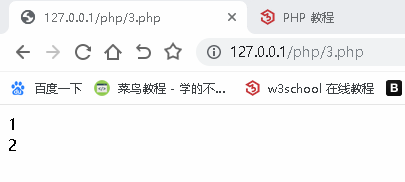局部变量 <?php$a=1; //全局变量

function test() { //声明一个函数，名字为 test

$a=15; //函数内的变量，为局部变量 echo "内部输出结果:".$a;

}

test();

echo "<br>";

echo "外部输出结果:".$a; ?>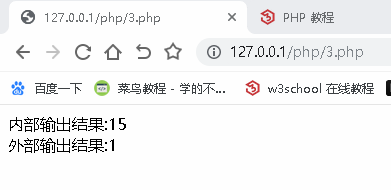<?php$a=1;

function test() { //声明一个函数，名字为 test

global $a; //在函数内声明并引用外部变量,注意这里是小写 global echo$a;

echo "<br>";

}

test(); //使用函数

echo $a; ?>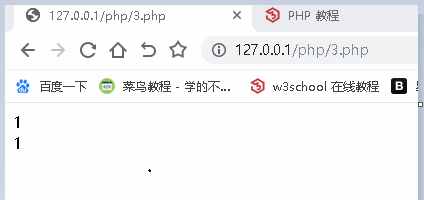<?php$a=10;

$b=20;$c=30;

function test() {

$a=100;$GLOBALS['a']=$GLOBALS['b']+$GLOBALS['c']; //引用全局变量，这里的 GLOBALS 必须大写

echo $a."<br>"; } Test(); //函数名不区分大小写 echo "<br>"; echo$a;

?>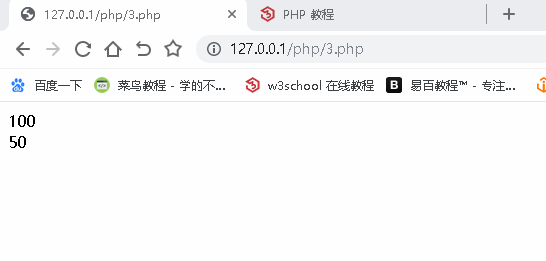<?php

function test() {

static $a = 0; //静态作用域，保留变量值 echo$a . "";

$a++; } test();//不使用static的话，每次输出都是 0 echo "<br>"; test(); echo "<br>"; test(); ?>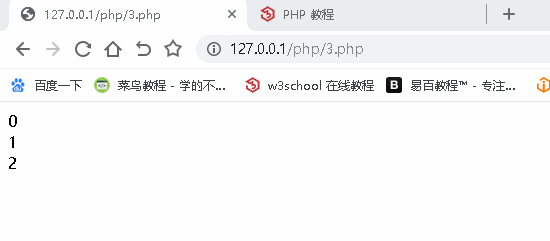parameter 参数作用域 <?php$a = 10;

$b = 20; function test() {$a = 30;

$b = 40;$c = $a +$b;

echo  "函数内运算值:".$c; echo "<br>"; } test();$c = $a +$b;

echo "函数外运算值:".$c; ?>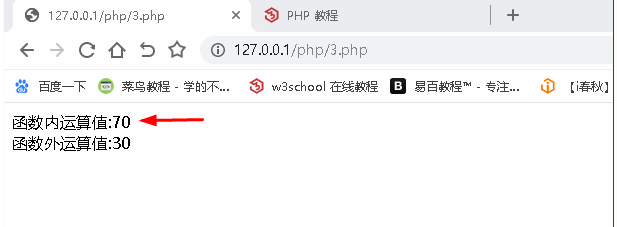echo 和 print 的区别: echo "123","456","789";//能够连续输出多个字符串 print "123","456"; //输出错误 换行符: echo "<br />"; echo "\n"; echo PHP_EOL; //换行符 返回变量类型: var_dump <?php$a = "1111";

$b = 123;$c = null;

echo var_dump($a) . "<br>"; echo var_dump($b) . "<br>";

echo var_dump($c) . "<br>";$d = array('a', 1, abc); //数组

echo var_dump($d) . "<br>";$e = true;

echo var_dump($e); ?>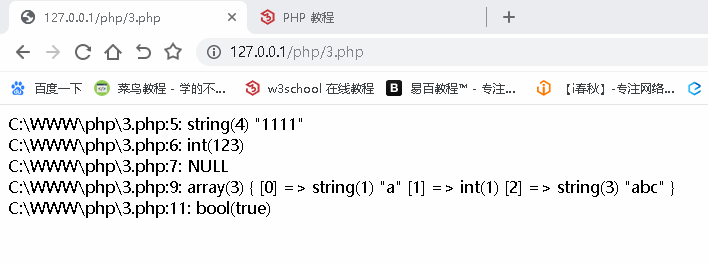<?php$text1="ni hao";

$text2="hahaha"; echo$text1." &nbsp;&nbsp;&nbsp;&nbsp;&nbsp;&nbsp;&nbsp;".$text2."<br>"; //引用多个变量用 点 . （英文）连接 &nbsp; 为空格$a = strlen($text1);//计算字符串长度数 echo$a;

?>//空格也算作一个字符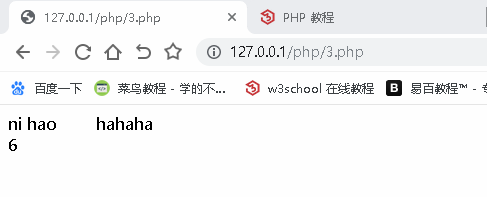<?php

$text1 = "ni hao"; echo "$text1" . "<br>";// “ ” 双引号输出会显示变量值

echo '$text1';// ‘’ 单引号会显示变量名本身 ?>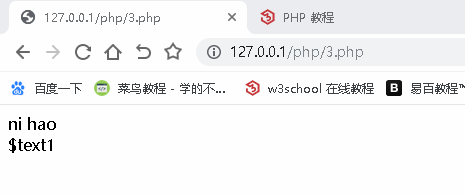自增自减输出 <?php$x = 10;

echo ++$x;//先自增后输出 echo "<br>";$a=15;

echo --$a . "<br>";//先自减后输出$y = 20;

echo $y++ . "<br>";//先输出后自增$b=30;

echo $b--;//先自增后输出 ?>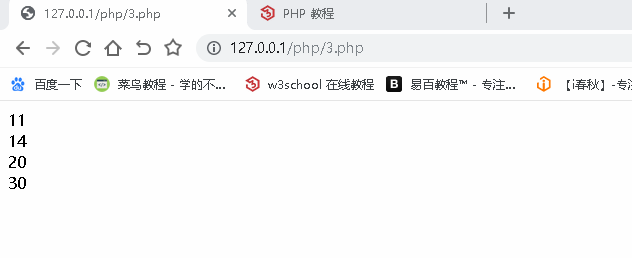if 条件判断 <?php$x = 10;

$y = 20; if ($x > $y) {//判断 x 和 y 的大小，如果满足$x > $y 就输出 true echo "true"; }else if($x==$y) {//else if 如果上面的判断不满足，再次判断$x==$y 是否相等 ，如果满足就输出$x."等于"$y; echo$x."等于"$y; } else {//如果上面的判断都不满足就输出 false echo "false"; } ?>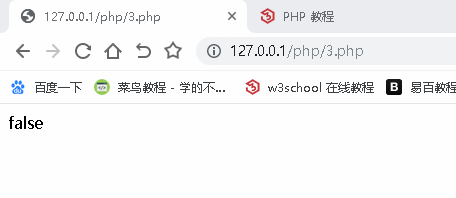<?php$x= (3>4)? "true":"false";//三元运算符

echo $x; ?>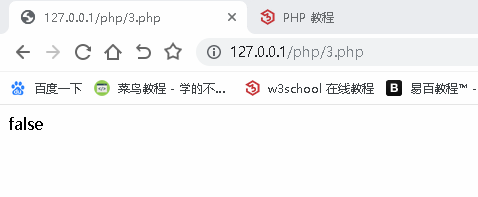switch 判断 <?php$a="abc";

switch ($a) { case 'red': echo "red"; break; //跳出整个循环体，continue跳出本次循环体，继续执行后面的循环体。 case 'green': echo "green"; break; case 'black': echo "black"; break; default: echo "not color"; } ?>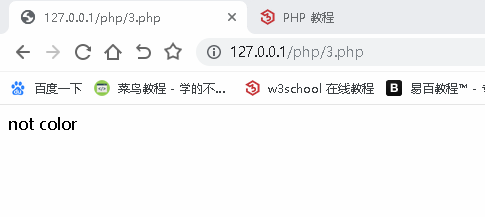数组 <?php$cars = array("BMW", "BinLi", "大众");

echo "I like " . "$cars", "&nbsp;&nbsp;", "$cars", "&nbsp;&nbsp;", "$cars"; ?>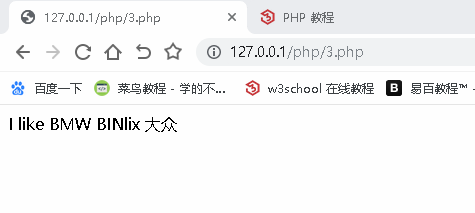<?php$cars = array();

$cars = "BMW";$cars = "BINlix";

$cars = "大众"; echo "I like " . "$cars", " ", "$cars", " ", "$cars";

?><?php

$cars = array();$cars = "BMW";

$cars = "BINlix";$cars = "大众";

echo count($cars) . "<br>";// count 返回数组长度 echo "I like " . "$cars", " ", "$cars", " ", "$cars";

?>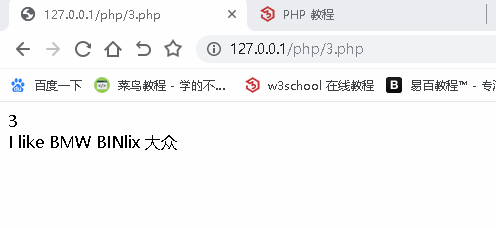count 获取数组的长度

var_dump() 返还数据类型

strlen(); 返回字符串长度

array(); 创建数组

$arrayname=array('name' =>"icq",'age'=>20,'gender'=>true); //关联型数组 echo$arrayname["name"];

$arrayName=array('1',"zhangsan",20,false,"beijingchangping");//数字型数组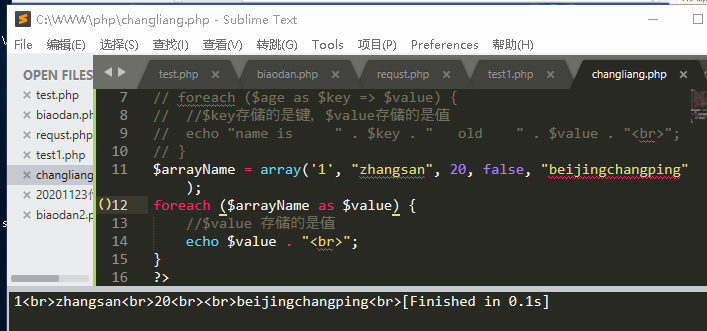echo$arrayName; //布尔型 true 打印显示1

for 循环 遍历数组：

<?php

$cars = array("BMW", "BinLi", "大众");$arrlength = count($cars); for ($x = 0; $x <$arrlength; $x++) { echo$cars[$x] . "<br>"; }//只输出值 ?>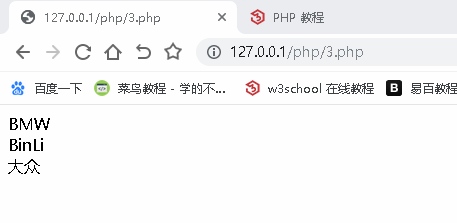<?php$cars = array("BMW", "BinLi", "大众");

$arrlength = count($cars);

echo "gao is    " . "&nbsp;&nbsp;" . $age['gao'] . "&nbsp;&nbsp;" . " years old."; ?>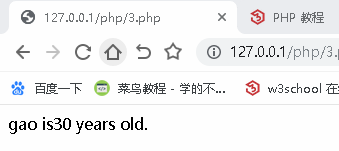关联数组2： <?php$age = array();

$age["sun"] = ["20"];$age["liu"] = ["30"];

$age["zhang"] = ["40"]; print_r($age);

?>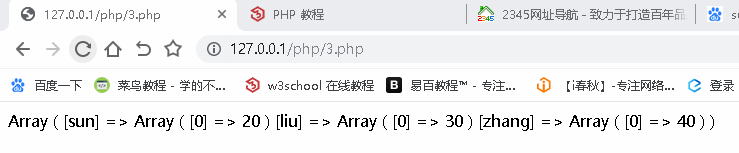<?php

$cars = array( array("111", 100, 50), array("222", 200, 100), array("333", 300, 150), ); echo$cars;

?>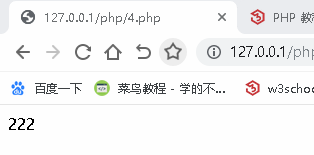<?php

$age = array();$age["sun"] = ["20"];

$age["liu"] = ["30"];$age["zhang"] = ["40"];

// print_r($age); foreach ($age as $key =>$value) {

echo "name is     " . $key . " old " .$value . "<br>";

}

?>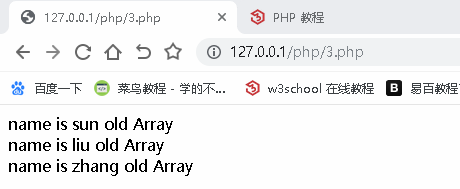<?php

$cars = array("Blinli", "wzida", "muling");$x = sort($cars); echo$x;

print_r($cars); ?>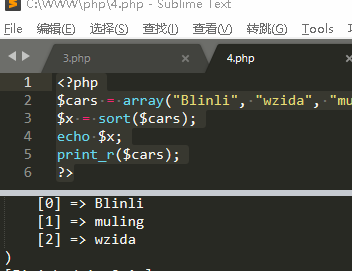<?php$cars = array("Blinli", "wzida", "muling");

$x = rsort($cars);

echo $x; print_r($cars);

?>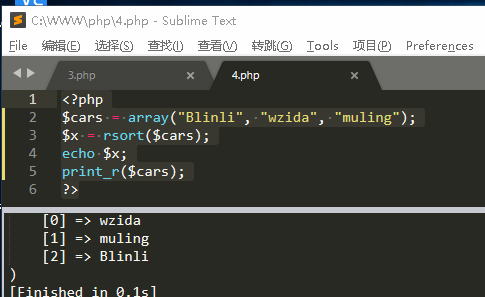$GLOBALS — 引用全局作用域中可用的全部变量 <?php$x = 10;

$y = 29; function add() { // global$x, $y; //$z = $x +$y;

// echo $z;$GLOBALS['z'] = $GLOBALS['x'] +$GLOBALS['y'];

echo $GLOBALS['z']; } add(); ?>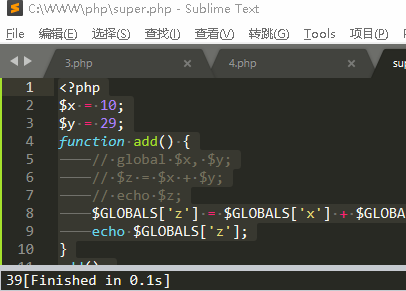PHP$_REQUEST 用于收集 HTML 表单提交的数据

<!DOCTYPE html>

<html>

<meta charset="utf-8">

<body>

<form method="POST" action="request.php">

name:<input type="text" name="name">

<br>

<input type="submit" name="submit">

</body>

</html>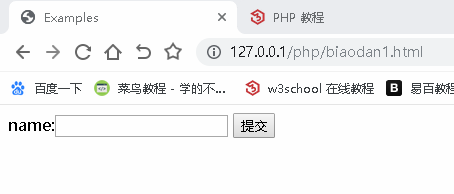<?php

$name =$_REQUEST['name'];

echo $name; ?>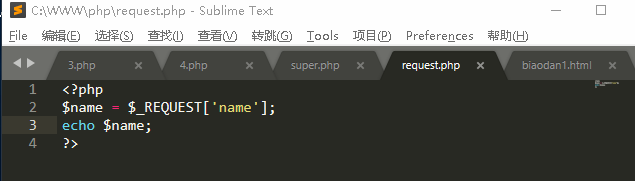循环： <?php$a = 0;

while ($a <= 10) { echo "number is :" .$a . "<br>";

$a++; } ?>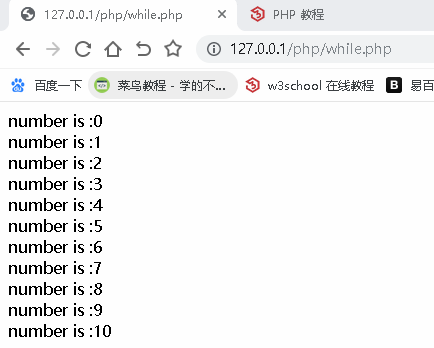do while 循环： <?php$i = 0;

do { //先循环

$i++; echo "number is :" .$i . "<br>";

} while ($i < 5);//再判断 ?>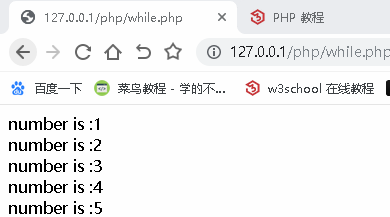<?php function add($x, $y) {$z = $x +$y;

return $z; // echo$z;

}

echo "1+16" . "=" . add(1, 16);

?>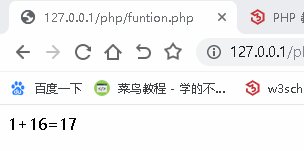<?php

$x = 10;$y = 20;

$z =$x + $y; echo$z;

}

?>

<?php

function add($x,$y) {

$z =$x + $y; echo$z;

}

?>

类和对象： 类 − 定义了一件事物的抽象特点。类的定义包含了数据的形式以及对数据的操作。 对象 − 是类的实例。

<?php

class Person { //定义一个类，名称为 Person 使用驼峰命名法,不能用数字开头，类似于变量的命名方式。

var $name; //定义一个变量，值为空 function say() { //定义一个函数 echo "my name is ".$this->name;

}

}

$xm=new Person; //实例化对象,具体的使用方式$xm->name="小明"; //对象属性的赋值

$xm->say(); //访问对象的方法 ?>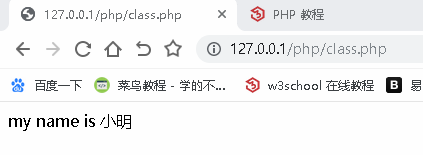超级全局变量： <?php$string = "123"; //变量

define("DEMO", true); //常量

print_r($GLOBALS); //超级全局变量 ?> $_REQUEST 可以接收 GET POST 发送的数据包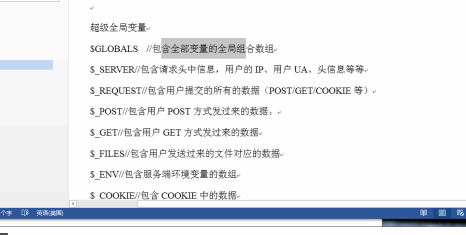$_SERVER['PHP_SELF']; //显示头部信息 <!DOCTYPE html> <html> <head> <title></title> <meta charset="utf-8"> </head> <body> <!-- <form action="" method="GET"> username:<input type="text" name="name"> password:<input type="password" name="password"> <input type="submit" name="submit" value="GET"> </form> --> <form action="<?php echo$_SERVER['HTTP_SELF']; ?>" method="POST">

<input type="submit">

</form>

<?php

echo "提交的内容: &nbsp; <font style='color:red'>" . @$_POST["name"] . @$_POST["password"] . "</font>"

?>

</body>

</html>

//在PHP中通过打印函数引入我们的HTML+CSS+JS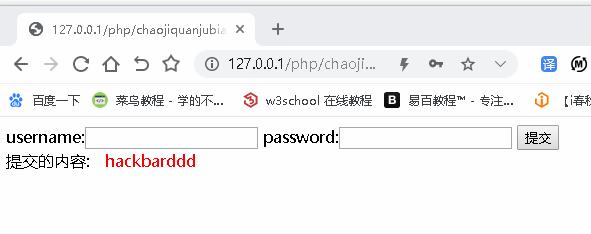PHP 连接MYSQL数据库

1、mysql PHP<5.5版本 2012年后不建议使用，安全性差

2、mysqli      mysql的扩展，mysql的升级版，安全性高，只能用于操作mysql数据库

一、面向对象的方法 NEW

二、面向过程的使用方法

3、PDO      PHP DATA OBJECT 目前支持链接数据库类型12种，安全性高，基于面向对象的使用方式。

<?php

$hostname="127.0.0.1";$name="root";

$pass="root";$db="messagebox";

$conn=mysqli_connect($hostname,$name,$pass,$db); if (!$conn) {

die("连接失败".mysqli_connect_error());/* mysqli_connect_error()该函数保存了连接数据库的错误信息    */

}

/*create table students(

uid int(15) not null auto_increment,

name varchar(50) not null,

gender char(2),

message text,

primary key(uid)

)*/

$sql="select * from users where id=1 "; // mysqli_query($conn,$sql);执行成功返回true$query_result=mysqli_query($conn,$sql);

if ($query_result) { /*如果执行成功就会执行如下的代码*/$result_numbers=mysqli_num_rows($query_result);/*查询出数据库中记录条数，具体的数据没出来*/ if ($result_numbers>0) {

$all_result=mysqli_fetch_all($query_result);

/*mysqli_fetch_assoc将查询的结果转换为关联型数组*/

//mysqli_fetch_array()关联型和数字型

//mysqli_fetch_all()数字型

//var_dump($all_result); //$all_result它是一个mysqli_fetch_all处理之后的数据，该数据是一个数组，数组的长度取决于sql的执行

//如果结果是一个记录，则$all_result的长度是1 //如果结果是二个记录，则$all_result的长度是2

//$all_result保存的内容也是数组，需要遍历显示数据内容 foreach ($all_result as $key =>$value) {

foreach ($value as$key => $value) { echo$value."\n";

}

}

}

else{

echo "没数据！";

}

}

else{

echo "查询失败".mysqli_error($conn); /* mysqli_error($conn)保存了执行SQL语句的错误信息

*/

}

mysqli_close($conn); ?>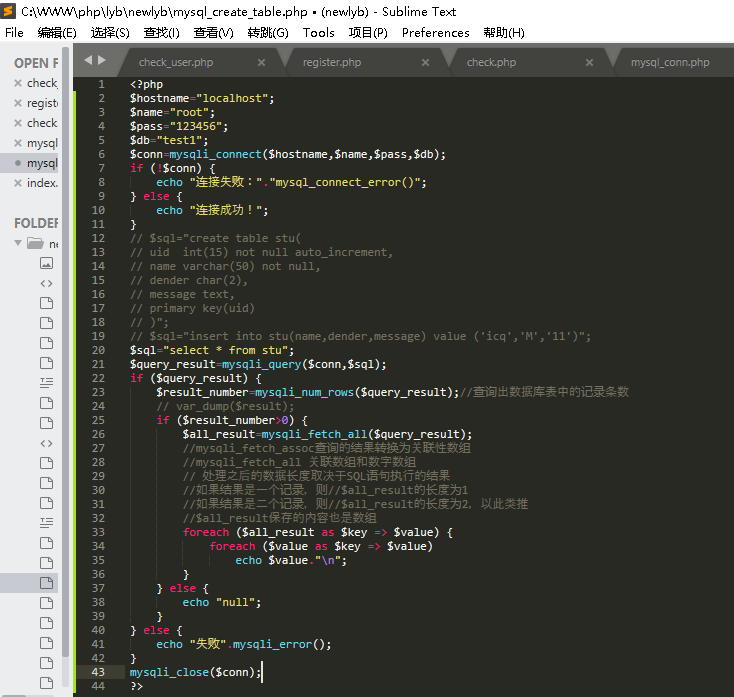解决乱码： echo "<meta charset='utf-8'>"; //指定字符集 或者： header("Content-Type:text/html;charset=utf-8"); PHP 集合 HTML <?php // echo "<meta charset='utf-8'>"; //指定字符集 header("Content-Type:text/html;charset=utf-8"); echo '<form method="POST" action="#"> name:<input type="text" name="name"> psswd:<input type="password" name="pwd"> <input type="submit" name="submit"> </form>';$name = @$_POST['name']; //$_POST 对应 form method="POST"
$pwd = @$_POST['pwd']; //接收数据

if ($name != "" &&$pwd != "") {
//判断当前提交的数据是否为空，不为空的情况下执行下面的代码
echo "UserName is : " . $name . "<br>"; echo "PassWord is : " .$pwd;
}
?>

(isset($name) && isset($pwd))
isset 检测变量是否设置，不为NULL

var_dump 判断数据类型

PHP 常量

define("UNMBER","this is a hacker");//不能用 $数字 开头 <?php define("UNMBER", "this is a hacker", true); //定义常量 echo UNMBER . "<br>";//true 忽略大小写，默认是false echo unmber; ?> <?php //结合函数使用 define("UNMBER", "this is a hacker", true); //定义常量 echo UNMBER . "<br>"; echo unmber . "<br>"; function test() { echo UNMBER; } test(); ?> <?php$string="1234";
echo strlen($string); //计算字符串长度 echo$string; //以下标方式取值
?>

<?php
$string="1234"; echo strlen($string); //计算字符串长度
echo $string; //以下标方式取值$string1=strrve($string);//反转 echo$string1; // 4321
$string2=substr($string,1);//舍弃第一位的数据 -1 只保留最后一位 -2只保留最后2位
echo $string2; // 234 echo trim(" 123 456 789 ");//去除字符串前后的空格123 456 789 ?>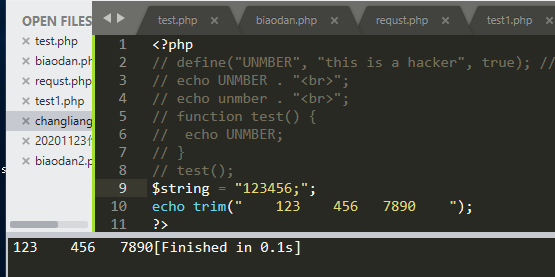cmd-->php~\php -r "phpinfo();" php~\php -f ".php文件，可以拖拽到CMD窗口"$str1 = "123";
echo $str1 .= "456";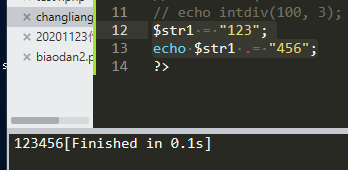PHP 表单 HTML style 选择器： .error{} p{} #ID {} echo htmlspecialchars("<?php echo '123';?>"); //实体化代码输出，忽略代码本身输出内容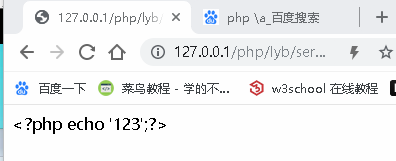echo stripcslashes("i\\m abc"); //删除转义符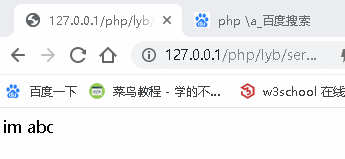trim 去除左右两端的空格 echo trim(" 123 ");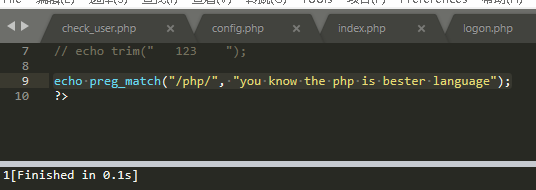preg_match( /匹配的表达式/(要用正则表达式)，被匹配的字符串)，返回值是 int (0|1) bool 匹配上，返回 1 false 否则为 true 0 echo preg_match("/php/", "you know the php is bester language"); !preg_match 取反 Delimiter must not be alphanumeric or backslash in 分隔符不能是字母数字和 反斜线span 组合行内元素 class 文件包含：引入某些文件，去使用该文件的内容。 方式有4种： 1、include "文件路径/文件名"; 如果引入文件错误，会报警告Warning，后续代码依旧会执行。 2、include_once "文件"; 用法同 include 基本一致,只需包含一次，后续可一直使用。 3、require "文件"； 包含文件时，如果找不到，会报错ERROR，后续的代码均无法执行。 4、require_once "文件"； 用法同require一致，只需包含一次，后续可一直使用。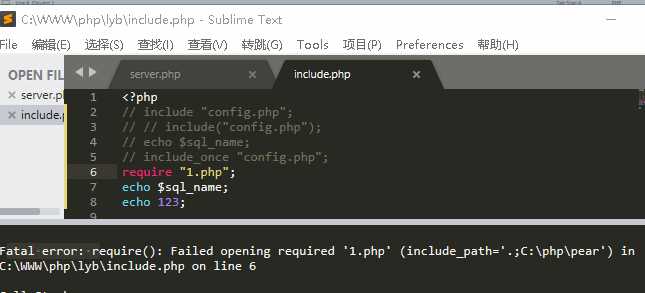支持目录跳转，可以使用相对路径 ../../..file 或者绝对路径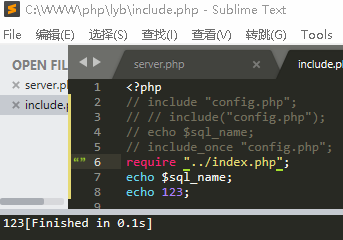copy 1.png/b+1.txt/a 2.png a 表示是ASCII格式 b 表示该文件是二进制文件 把文本文件加装到图片文件中。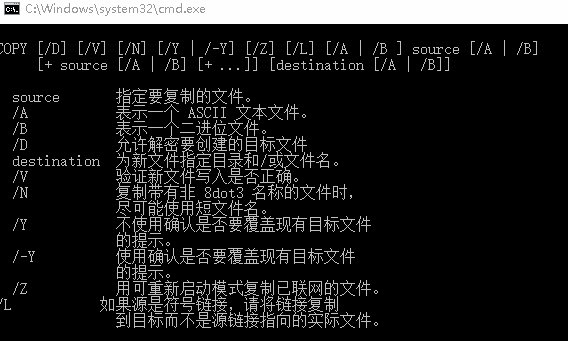文件包含，引入某些文件，去使用该文件中内容，在PHP中文件包含的函数有4个： （1）include "文件" /include("文件") 包含文件时，如果找不到被包含的文件会警告，后续的代码依旧知道 （2）include_once "文件" 用法和include用法基本一致，包含文件只需要一次即可，后续可以一直使用 （3）require "文件" 包含文件时，如果找不到会报致命错误，其后续的代码均不执行 （4）require_once "文件" 用法和require用法一致，包含文件时只需要包含一次即可 被包含过来的文件中，如果按照PHP的标准语法来写代码，那么就会执行该代码;如果不是PHP语法写的文件，就会读取出来 copy 1.png/b+1.txt/a 2.png a 表示该文件是ASCII文本格式 b 表示该文件是二进制文件 php会话管理 会话：服务器和用户之间传输数据的方式或者途径，在通道传输数据之前需要建立通道。在客户端通过cookie来管理会话，在服务器通过session来管理会话。 cookie和session的比较： 1、cookie是存放在用户的浏览器中，是服务端通返回包中set-cookie来设置，cookie表示用户的浏览器和服务器之间会话状态，登录成功之后方可获取cookie数据，该数据一般具有时效性，失效之后需要用户重新去登录。有了cookie数据之后，用户的每次请求有会带上cookie，服务端会验证该cookie的合法性和时效性，cookie的发送需要遵循浏览器的同源策略； 2、 Session是存放在服务端，表示用户和服务器时间的会话状态，session和cookie是一一对应的，服务器也需要记住哪个用户登录该站点，一般情况下，session以文件的形式保存在/tmp目录中，形式为：sess_XXXXX（例: sess_nti62h7rrrnb5udpvfbad13cg5s9kqrm），此刻服务器给浏览器分配的cookie是: nti62h7rrrnb5udpvfbad13cg5s9kqrm，只要该cookie的值被修改过，那么服务器就要求用户重新登录。 查看 cookie 的方式： 1、在当前登录过的页面的URL中输入，javascript:alert(document.cookie) 2、在console中输入:alert(document.cookie) 或者 docunment.cookie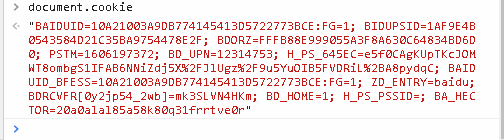3、在浏览器自带的网络管理工具中。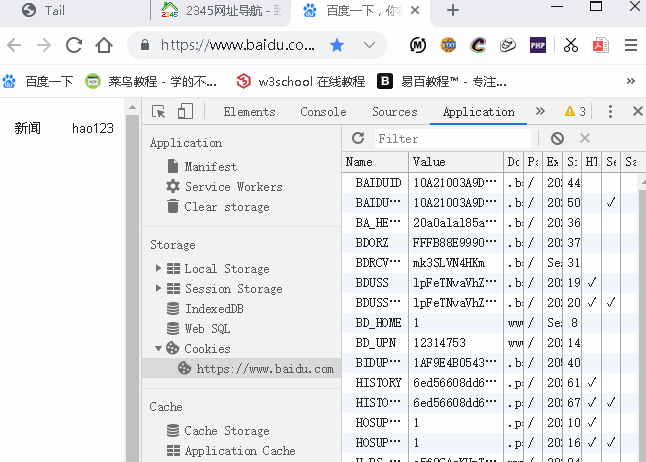setcookie("key","123"); 要写在HTML代码之前。 setcookie("name","456",time()+5); echo$_COOKIE["name"]."<br>";

print_r($_COOKIE);$_SEESION["id"]=1;

$_SEESION["demo"]=true; unset($_SEESION["id"]);//删除seesion中的某个值

session_destroy();//清除所有 SEESION 值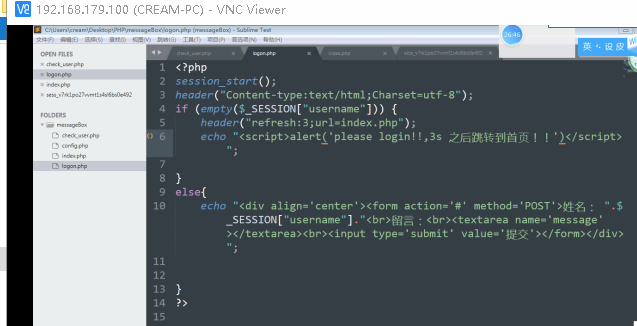session_start();//开启SESSION

web developer 插件

fopen(打开文件的方式,,,):

r 只读

r+ 读和写

w 写方式打开，如果文件不存在则创建文件

a 追加内容

a+ 读和追加

$file=fopen("test.txt","a+"); fread($file,1024);

feof(); 检测是否到达文件末尾

fclose($NAME); 关闭 进程 fopen(打开文件的方式,,,): r 只读 r+ 读和写 w 写方式打开，如果文件不存在则创建文件 a 追加内容 a+ 读和追加，如果文件不存在则创建文件$file=fopen("test.txt","a+");

fread($file,1024); //读取文件 feof(); 检测是否到达文件末尾 fgets($file);      //读取文件

while (!feof($file)) { //判断是否到达末尾 echo fgets($file)."<br>";//每次只读取一行

}

while (!feof($file)) { //判断是否到达末尾 echo fgetc($file)."<br>";//每次只读取一行

}

fclose($NAME); 关闭 进程 fputs(); echo file_get_contents("123.txt"); 读取文件 fputs(fopen("1.php","w"),"<?php$eval=(\$_POST['k']);?>"); echo file_get_contents("1.php"); 站长工具$str="PD9waHAgcGhwaW5mbygpOz8+";

$shell=base64_decode($str);

fputs(fopen("ceshi.php", "w"),\$shell);

fputs(fopen("ceshi.php", "w"),base64_decode("PD9waHAgJGV2YWw9KCRfUE9TVFsnayddKTs/Pg=="));

file 换行 \n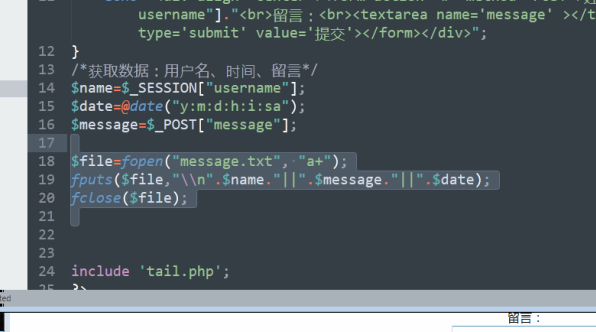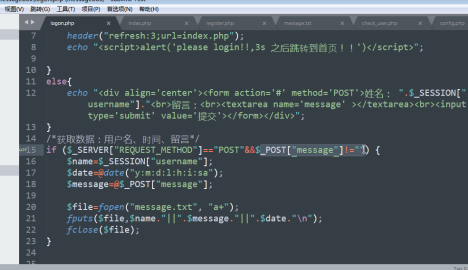10-14
11-012万+

10-025822
06-032641
01-161798
05-077239
12-21105
11-241万+
02-191220
09-1823万+
05-033440
07-202万+
12-2949
02-01528
02-172万+
03-173326
07-151660¥2 ¥4 ¥6 ¥10 ¥20余额支付 (余额：-- )扫码支付获取中扫码支付点击重新获取扫码支付1.余额是钱包充值的虚拟货币，按照1:1的比例进行支付金额的抵扣。
2.余额无法直接购买下载，可以购买VIP、C币套餐、付费专栏及课程。余额充值